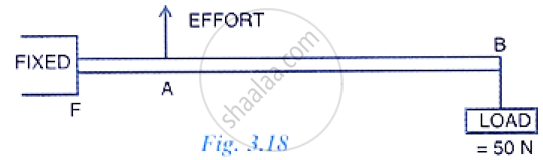Share
Notifications

View all notifications
Books Shortlist
Your shortlist is empty

# Fig 3.18 Below Shows the Use of a Lever. (A) State the Principle of Moments as Applied to the Above Lever. (B) Give an Example of this Class of Lever. If Fa = 10 Cm, Ab = 500 Cm Calculate: - ICSE Class 10 - Physics

Login
Create free account

Forgot password?

#### Question

Fig 3.18 below shows the use of a lever.(a) State the principle of moments as applied to the above lever.
(b) Give an example of this class of lever.
(c) If FA = 10 cm, AB = 500 cm calculate: (i) the mechanical advantage and (ii) the minimum effort required to lift the load.

#### Solution

(a) The principle of moments: Moment of the load about the fulcrum=moment of the effort about the fulcrum
FB × Load = FA × Effort

(b) Sugar tongs the example of this class of lever.

(c) Given: FA = 10 cm, AB = 500 cm, BF = 500 + 10 = 510 cm.
The mechanical advantage
M.A = "AF"/"BF"=  10/510= 1/51
The minimum effort required to lift the load
Effort = "Load "/"M.A"=50/(1/51) = 2550 N

Is there an error in this question or solution?

#### APPEARS IN

Solution Fig 3.18 Below Shows the Use of a Lever. (A) State the Principle of Moments as Applied to the Above Lever. (B) Give an Example of this Class of Lever. If Fa = 10 Cm, Ab = 500 Cm Calculate: Concept: Concept of Lever.
S